## 5.2 Formal Presentation

In this section, we briefly outline the formal presentation of dependency grammar presented in [Duc99a]. The basic idea is that each node of the dependency tree must be assigned a lexical entry from the lexicon, and that certain principles of well-formedness must be verified. For example, the lexical entry stipulates a valency, and the node must have precisely the outgoing dependency edges that realize this valency.

A dependency grammar is a 7-tuple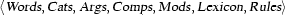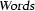a finite set of strings notating fully inflected forms of words.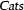a finite set of categories such as n (noun), det (determiner), or vfin (finite verb).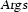a finite set of (what we call) agreement tuples such as <masc sing 3 nom>.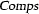a finite set of complement roles, such as subject or zu_infinitive.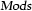a finite set of modifier roles, such as adj (adjectives), disjoint from. We writefor the set of all types of roles.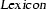a finite set of lexical entries (see below).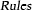a finite family of binary predicates, indexed by role labels, expressing local grammatical principles: for each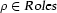, there is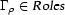such thatcharacterizes the grammatical admissibility of a dependency edge labeledfrom mother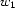to daughter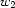.

A lexical entry is an attribute value matrix (AVM) with signature: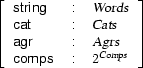and we write attribute access in functional notation. Ifis a lexical entry, then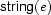is the full form of the corresponding word,is the category,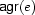is the agreement tuple, and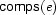the valency expressed as a set of complement roles.

### 5.2.1 Dependency Tree

We assume given a set of nodes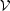which for simplicity we identify with the set of integers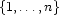, and a set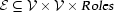of labeled directed edges between these node.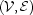is a directed graph, in the classical sense, with labeled edges. We restrict our attention to finite graphs that are also trees.

A dependency tree is then defined as a pair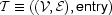of a tree as stipulated above and a functionmapping each node into a lexical entry in.

### 5.2.2 Well-Formedness Principles

Not all dependency trees as described above are grammatically admissible. We now describe the conditions of admissibility, aka well-formedness.

While we have identified nodes with integers, we still prefer to write(and often justor) instead ofto remind the reader that they correspond to words and to distinguish them from other occurrences of integers that have no such interpretation. We also writeto represent an edge labeledfrom motherto daughter.

First, any complement required by a node's valency must be realized precisely once:Second, if there is an outgoing edge from, then it must be labeled by a modifier role or by a complement role in's valency: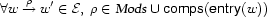Third, whenever there is an edge, then the grammatical conditionfor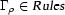must be satisfied in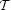: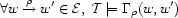Denys Duchier
Version 1.2.0 (20010221)